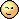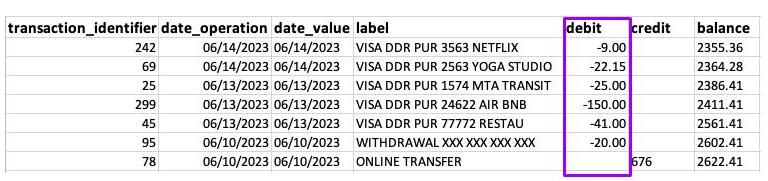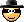Last updated on 4/28/21

## Discover the Four Variable TypesVariables come in two types, each of which is subdivided into two groups. Hence, four variable types.

These are variables that take a numerical value (in other words, a number!)—provided this numerical value represents a mesurable quantity and mathematical operations can be applied to it.

For example, if you add together all of the expense amounts in your bank statement, you will get the total amount you have spent.This makes sense. It’s an amount of money. If you were to add up the amounts of each transaction, you would know that you spent \$267.15 between 06/10/2023 and 06/14/2023.

However, a transaction identifier, despite being numerical, is not a quantitative variable. It makes no sense to add up all of your transaction identifiers as the identifier is not a measureable quantity.  I shouldn’t be telling you this (it will spoil the surprise!), but an identifier is a qualitative variable (shhhh, you didn’t hear it from me!).

Discrete variables have a countable number of values between any two values. Think of a digital clock. A discrete value for time could be 5:34pm. This is discrete because the next value is 5:35pm.

Continuous variables have an infinite number of values between any two values. This time, think of an analog clock. We could have hours and minutes, but we can also include seconds, milliseconds, microseconds and so forth. In other words, there are no breaks between two values.

A qualitative variable is any variable that is not quantitative . The values a qualitative variable can take are called classes, or categories. Categories are expressed in literal form (by word, phrase, or code) or by numerical codes to which mathematical operations cannot be applied.

A variable is ordinal if its categories can be ordered. For example, traditional exam grades (A, B, C) are ordinal because A is considered to be a better score than B, which is considered to be a better score than C. The transaction identifier is a nominal (or categorical) variable, because transaction no. 1 is not necessary “less” or "more" than transaction no. 40 (this is assuming the identifiers are not necessarily ordered by transaction date)

And there you have it: now you know everything!Oh, wait... the so-called dichotomous variables. We have yet to talk about those. Well, these are qualitative variables that take on only one of two categories such as  `yes/no`  or   `true/false`  . These are also called binary variables, or Booleans (abbreviated “bool”).

Computer systems store dates in the form of integers called timestamps. Timestamps count the number of seconds (and sometimes milliseconds) that have ticked away since January 1, 1970. For example, the date of September 23, 2020, is coded as follows by the timestamp:  `1600819200`  .